In this blog we will be discussing bivariate analysis through Visualization in which we will be taking care of two or more variable at the same time. Through this we can see the correlation, interaction and relationship between two variables.  For this we will be using python internal dataset “tips”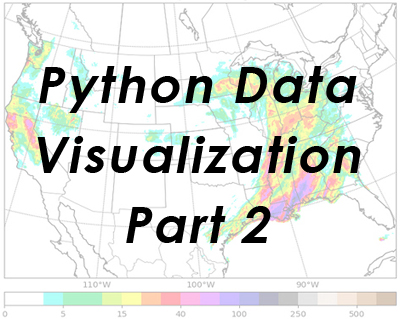Importing the libraries

import pandas as pd

import numpy as np

import seaborn as sns

importmatplotlib.pyplot as plt

%matplotlib inline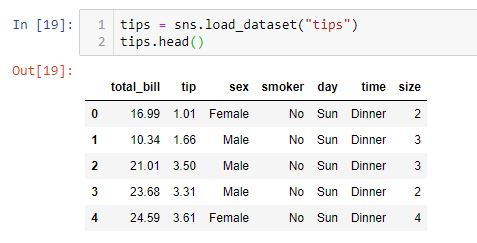Importing the dataset

Bar Plot

Bar plots uses bars to compare data between different categories. It can be horizontally or vertically aligned.

sns.set(rc={‘figure.figsize’:(10,10)})

sns.set(style=”whitegrid”)

ax = sns.barplot(x=”day”, y=”total_bill”,hue=”sex”, data=tips,estimator = sum,color=”salmon”)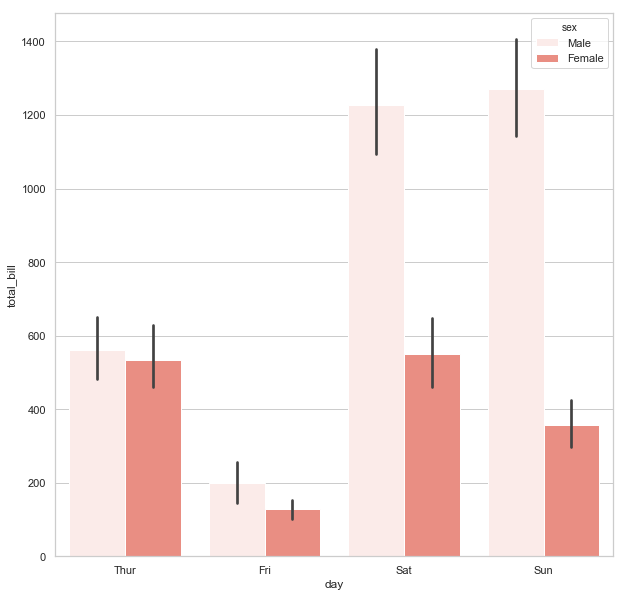Count Plot

It is used to plot the frequency of each unique observation in a categorical variable.

# ‘hue’ is used to as an additional variable in the existing graph

sns.countplot(tips.sex, hue=tips.smoker,color=”salmon”)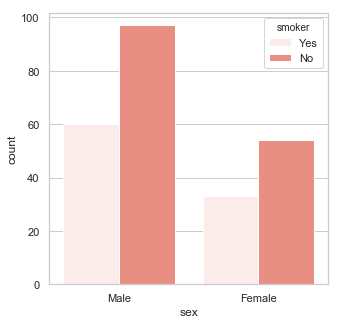Strip Plot

Strip Plot is used to see the distribution of a continuous variable with respect to different levels of a categorical variable.

sns.stripplot(tips[‘smoker’],tips[‘total_bill’] , jitter=True,color=”salmon”)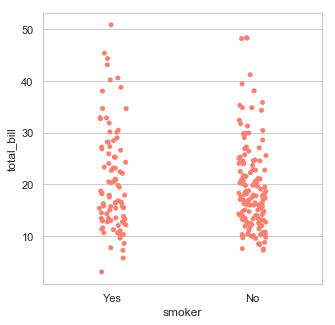Pair Plot

A pair plot is used to see the pairwise relationship between the variables in a dataset.

sns.pairplot(tips,hue=’smoker’)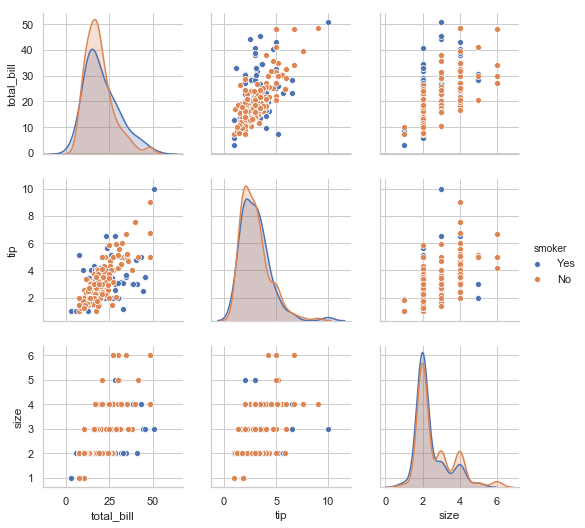Conclusion: – In this blog we have discussed different visualization that can be used for two or more variables; through these you can determine correlation and association between variables.